C. The Friedmann Models

To describe the expansion of the universe one must use the RWM along with the Einstein equations,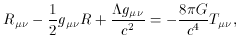(8)

to determine the equations of motion. For reference, a summary of the metric coefficients, the Christoffel Symbols, and the Ricci Tensor components are presented in [13, Chapter 15]. Note that in this book the scale factor a(t) is written R(t).

Before proceeding any further, an appropriate stress-energy tensor must be provided. This is the difficult part of the process. The composition of the known universe is a very controversial topic. The standard procedure is to consider simplified distributions of mass and energy to get an approximate model for how the universe evolves.

At this point, units are chosen such that the speed of light, c is set equal to unity. This gives the simplification that the energy density,is equal to the mass density,using,=c2 =. This also allows mass and energy to be considered together, which is in the spirit of the stress-energy tensor. The mass/energy density will be referred to as the energy density for the remainder of this paper. The stress-energy tensor may be given as: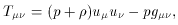(9)

where p is the pressure,is the density, and uµ is the four-velocity.

At the earliest epoch of the universe, the contribution of photons to the energy density would have been appreciable. However, as the universe cooled below a critical temperature, allowing the photons to decouple from baryonic matter, the photon contribution became negligible. Thus, it is easier to consider different energy distributions for different epochs in the universe. The massive contribution to the energy density is usually referred to as the Baryonic contribution, since baryons (protons, neutrons, etc.) are significantly more massive than leptons (electrons, positrons, etc.) and leptons can therefore be disregarded as a major contributing factor to the total energy density. There is also the contribution of vacuum energy, which enters the Einstein equations through the cosmological constant,.

For each type of contribution, there is a corresponding density,. The total density can be expressed as the sum of the different contributions as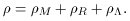(10)

Furthermore, assuming that one is dealing with a homogeneous and isotropic fluid, the density can be related to the pressure by a simple equation of state (see Table 1),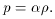(11)

 DensityPressure pEpochR/3 1/3 Radiation DominatedM 0 0 Matter Dominated (Non-relativistic Dust)--1 Vacuum Domination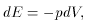which may be rewritten as,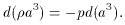(12)

Relating (11) and (12) gives,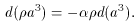Using the product rule,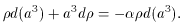Which can be integrated,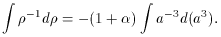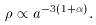(13)

In the Radiation epoch, where the energy density due to photons was appreciable (from about t = 0 to approximately 300,000 years after the Big-Bang ), the density due to massive particles can be neglected. The pressure is found to be equal to a third of the density, and we have a value of one-third for, soR ~ a-4 .

Following this epoch, the Matter Dominated epoch can be modeled after a `dust' that uniformly fills space. Because the temperature of the universe had fallen to around 3000 K, most of the particles had non-relativistic velocities (v << c). This corresponds to a negligible pressure andis therefore zero,~ constant .

The last case to consider is that of the vacuum energy. If the cosmological constant is indeed nonzero, this form of energy density will dominate. For this relation, the pressure is commensurate with that of a negative density. This would imply a value of -1 for, soM ~ a-3 . These results are summarized in Table 1.

Given an expression for the energy-momentum tensor, one can now proceed to find the equations of motion. The metric coefficients follow from the Robertson Walker line element, which is given by equation (4). Using these coefficients one can obtain the expression for the left side of the Einstein equations (8). Thus, from the Einstein equations one derives the Friedmann equations in their most general form: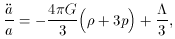(14)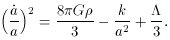(15)

Apparently, if the universe is in a vacuum dominated state p = -, (14) indicates the universe will be accelerating. This important conclusion will be the most general requirement for an inflationary model.

Now is the time to introduce a bit of machinery to make our calculations more tractable. Recall that the Hubble constant, H(t), is defined as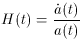(16)

Next, one defines the Deceleration parameter (named for historical reasons) as,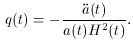(17)

To realize how this term arises, consider the Taylor expansion of the scale factor, about the present time, t0,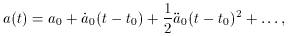where the sub-zeros indicate the terms are evaluated at the present. Using equations (16) and (17), this becomes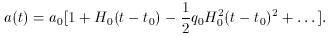(18)

Remembering that the crux for obtaining Hubble's Law (1) was measuring the luminosity distance, it is of interest to consider this calculation quantitatively. The flux F (energy per time per area received by the detector) is defined in terms of the known luminosity L (energy per time emitted in the star's rest frame) and the luminosity distance dL.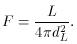(19)

The luminosity distance must take into account the expanding universe and can be written in terms of the redshift, z as ,(20)

where a0 is the present scale factor and r is the comoving coordinate that parameterizes the space. Hubble used the measured flux and the known luminosity to find the distance to the objects he measured. The distance can then be compared with the known redshift of the object using (20) and the velocity can be approximated. However, r in (20) is not a observable and it is of interest to examine the great amount of estimation that must be used to derive the desired result analytically.

Dividing (18) by a0 and making use of (7) yields,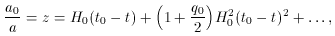(21)

which can be solved for (t0 - t),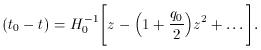(22)

One can also expand (6) in a power series,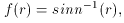(23)

where re has been replaced by r (for simplicity) and sin n-1 (r) is defined as sin1 (r) for k = 1, sinh-1 (r) for k = -1 and r for k = 0. So to lowest order, (6) can be estimated as r, and the l.h.s. of (6) can be estimated as,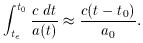(24)

Using the approximation from (23) and the above result we have,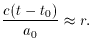Substitution of t - t0 from (22) and keeping only lowest order terms yields,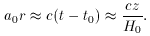At small redshift, z << 1 one finds zv/c. Thus, making this final approximation one obtains,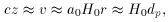(25)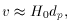(26)

where dp is the physical distance. Thus, we have obtained Hubble's law (1) as an approximation. This derivation reflects the reason that the law only holds locally. The number of approximations that were needed to proceed was appreciable. Furthermore, one finds that this law deviates significantly at large z as one would expect.

For a matter dominated model, one finds the exact Hubble relation to be given by ,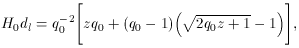(27)

which depends on the deceleration parameter, q0, which in turn relies on the curvature and the total mass density of the universe.